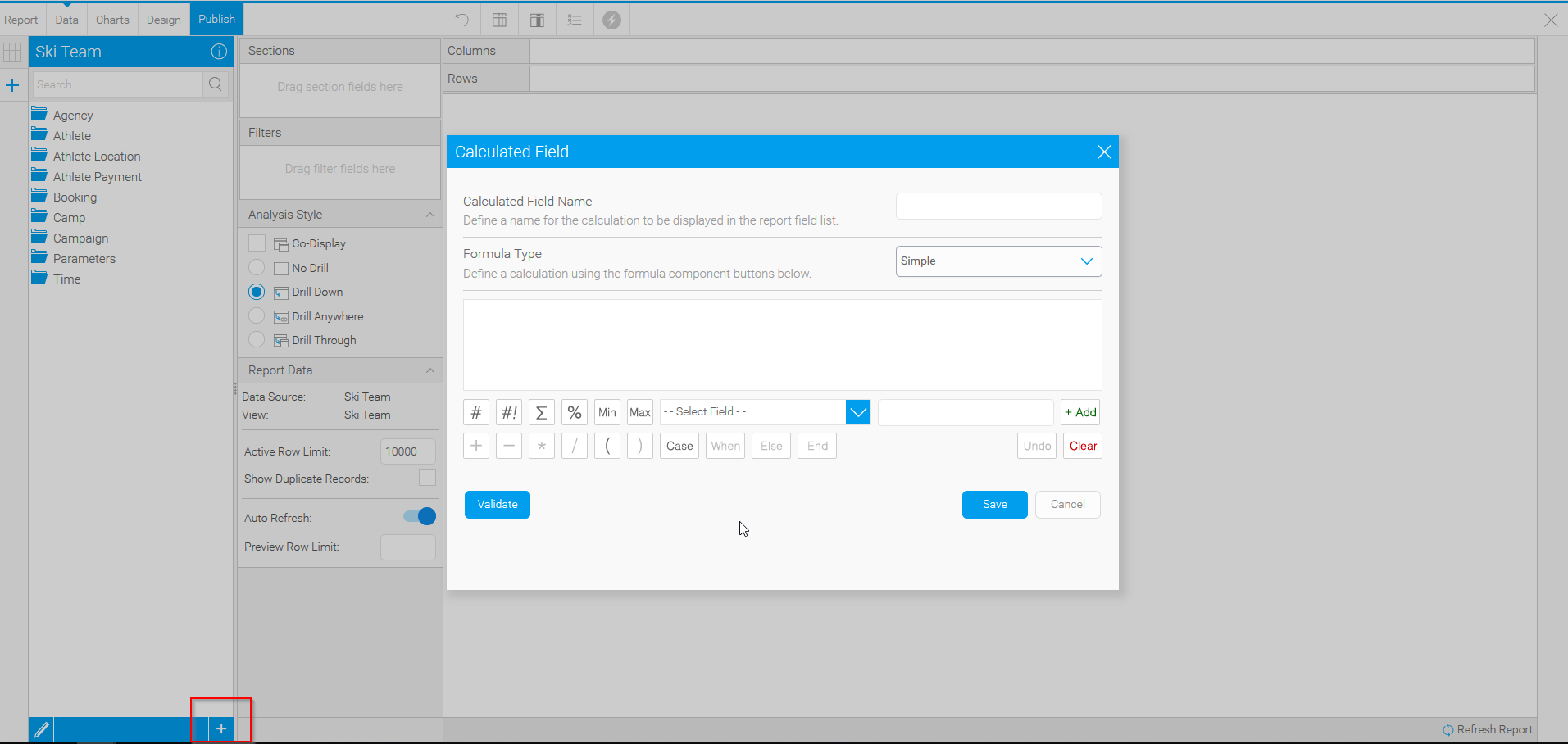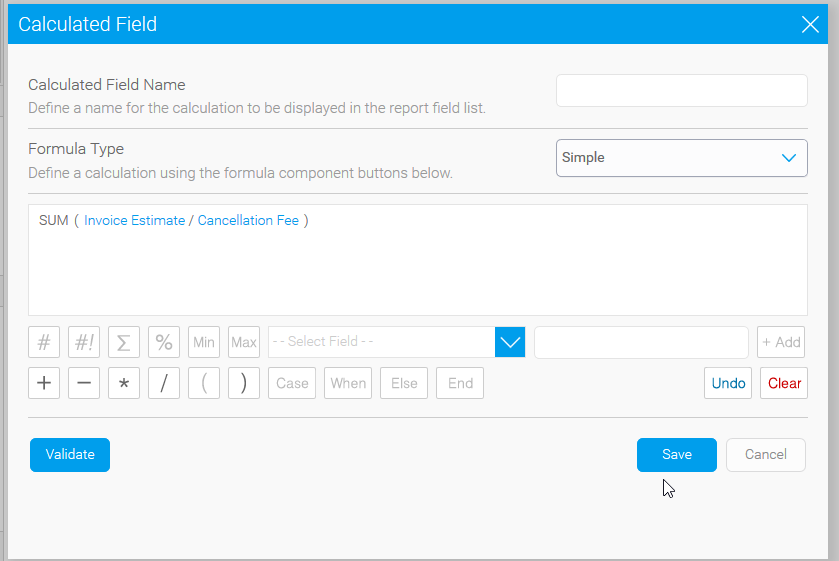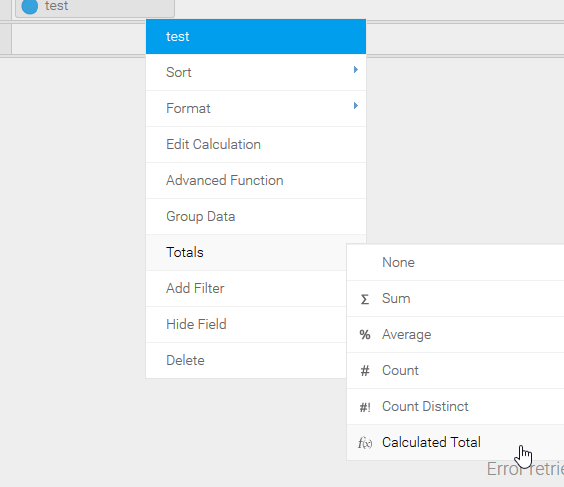# Numerator/Denominator - incorrect value in Numeric Display

Austin Hart shared this question ago

My calculation is SUM(Numerator*1.0)/SUM(Denominator*1.0). You can do the same calculation with division of columns.

Do the math:

568973/393468 = 1.44

However, when I pull my calculation over - an aggregation type must be selected and all of the aggregation types give the wrong value. If I select average (which is the closest) - it gives me 1.49. It's doing an average of averages.

I don't want to use any of those aggregation types - How do I get it to display 1.44 not 1.49?1

Hello Michelle,

This sounds like a perfect case for a Calculated Field and Calculated totals.

With a Calculated Field you will be able to generate a field with your desired calculation and then use the aggregation option Calculated Total to maintain your calculation.

To create a calculated field click the (+) in the bottom of the chart builder:Then the above window will pop up where you will input your calculation:Then you will have a new folder on the left side of the report builder containing calculated fields. Now when we go to the aggregation options of this calculated field we have the option to use calculated totals. This option should give you what you are looking for:Please let me know if this information was useful or if I completely misunderstood the question.

Thanks,

Jared1

That works fine if you are calculating a number all by itself - with no details. But if you want to include it at the bottom of a detail report or do this calc on a time series chart, it gives you the wrong number. I found one similar, after I wrote this, and it is a known defect of giving the incorrect answer. You can close this one for now. The other one is still open.

Thank you1

Hello Michelle,

Thank you for the update.